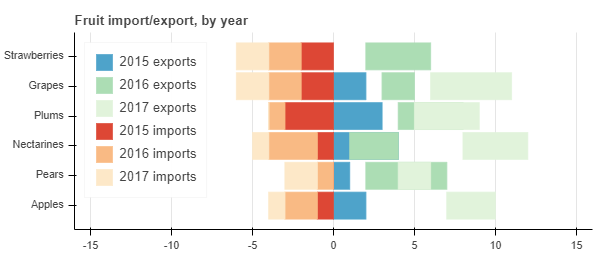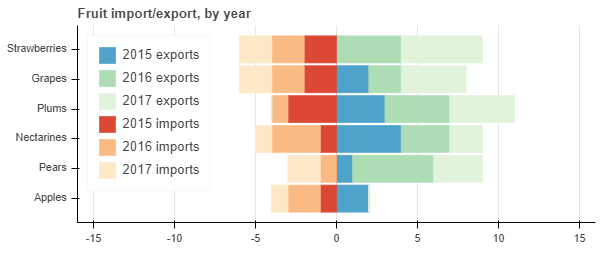# `hbar_stack` with different columns for the `y` attribute

I want to be able to plot a stacked horizontal bar chart where some of the columns are missing. Here’s an example from the docs that I’ve modified to show what I mean:

``````from bokeh.io import show
from bokeh.models import ColumnDataSource
from bokeh.palettes import GnBu3, OrRd3
from bokeh.plotting import figure

fruits = ['Apples', 'Pears', 'Nectarines', 'Plums', 'Grapes', 'Strawberries']
years = ["2015", "2016", "2017"]

exports = {'fruits1' : fruits[:-1],
'fruits2' : fruits[1:],
'2015'    : [2, 1, 4, 3, 2],
'2016'    : [5, 3, 4, 2, 4],
'2017'    : [3, 2, 4, 4, 5]}
imports = {'fruits' : fruits,
'2015'   : [-1, 0, -1, -3, -2, -2],
'2016'   : [-2, -1, -3, -1, -2, -2],
'2017'   : [-1, -2, -1, 0, -2, -2]}

p = figure(y_range=fruits, plot_height=250, x_range=(-16, 16), title="Fruit import/export, by year",
toolbar_location=None)

p.hbar_stack(years, y=['fruits1', 'fruits2', 'fruits1'], height=0.9, color=GnBu3, source=ColumnDataSource(exports),
legend=["%s exports" % x for x in years])

p.hbar_stack(years, y='fruits', height=0.9, color=OrRd3, source=ColumnDataSource(imports),
legend=["%s imports" % x for x in years])

p.ygrid.grid_line_color = None
p.legend.location = "top_left"
p.axis.minor_tick_line_color = None
p.outline_line_color = None

show(p)
``````

This produces the following plot:You can see that the `stack` objects created by the successive calls don’t take into account the change in the column name given in the list passed as `y` in the call to `hbar_stack`.

Obviously the solution in this example is to pad with zeros in the right places, but in my application this is not easy because the offset is arbitrarily large. Is there a way around this with Bokeh?

I’ve also posted this on StackOverflow.

I’ve also posted this on StackOverflow.

Respectfully, please don’t do this. It increases the burden of people already overwhelmed trying to help with support questions.

I don’t think there is any clean way to get `hbar_stack` to do this, since it is explicitly designed to stack up columns that align. And if fact, what you are actually telling Bokeh to stack in the code above is categorical values (i.e. you are asking for string category values to be summed), which does not really make sense. If you want to stack things that do not align then I think you will either need to zero-pad as you suggest, or else compute the stacked coordinates yourself according to the rule you want.

OK. Sorry about the double post; I’ll avoid that next time. If I come up with a solution, where should I post it?

I guess I prefer here but more than anything I just prefer it be one place and not many1 Like

I ended up doing something like this:

``````from bokeh.io import show
from bokeh.models import ColumnDataSource
from bokeh.palettes import GnBu3, OrRd3
from bokeh.plotting import figure
import numpy as np

def get_fruits_by_key(d):
"""Get all the distinct values for the columns of the dict with names containing the string s.

To be preserved, each entry must have a corresponding nonzero quantity.

Args:
d (dict): dict to be converted to ColumnDataSource.
Returns:
(np.ndarray): array of unique values from the columns in ascending order.
"""
values = set()
for key in d:
if '_fruits' in key:
for i, price in enumerate(d[key]):
if d[key[:-7]][i]:

return np.array(sorted(values))

def collect_fruits(d):
"""Combine the fruit columns in one, and update the quantity columns to match.

Args:
d (dict): dict to be converted to ColumnDataSource.
"""
fruits = get_fruits_by_key(d)

to_delete = set()

for key in d:
if key.endswith('_fruits'):
new_qty = []
for fruit in fruits:
if fruit in d[key]:
# the fruit is already in this fruit column and its quantity can be kept
new_qty.append(d[key[:-7]][d[key] == fruit])
else:
# the fruit is not in d, so there's no quantity there
new_qty.append(0)
d[key[:-7]] = np.array(new_qty)

for key in to_delete:
del d[key]

d['fruits'] = fruits

def main():
"""Run a test case."""
years = ["2015", "2016", "2017"]
exports = {
'2015_fruits': np.array(['Apples', 'Pears', 'Nectarines', 'Plums', 'Grapes']),
'2016_fruits': np.array(['Pears', 'Nectarines', 'Plums', 'Grapes', 'Strawberries']),
'2017_fruits': np.array(['Pears', 'Nectarines', 'Plums', 'Grapes', 'Strawberries']),
'2015': np.array([2, 1, 4, 3, 2]),
'2016': np.array([5, 3, 4, 2, 4]),
'2017': np.array([3, 2, 4, 4, 5])
}
imports = {
'fruits': ['Apples', 'Pears', 'Nectarines', 'Plums', 'Grapes', 'Strawberries'],
'2015': [-1, 0, -1, -3, -2, -2],
'2016': [-2, -1, -3, -1, -2, -2],
'2017': [-1, -2, -1, 0, -2, -2]
}

collect_fruits(exports)

p = figure(y_range=imports['fruits'], plot_height=250, x_range=(-16, 16),
title="Fruit import/export, by year", toolbar_location=None)

p.hbar_stack(years, y='fruits', height=0.9, color=GnBu3, source=ColumnDataSource(exports),
legend=["%s exports" % x for x in years])

p.hbar_stack(years, y='fruits', height=0.9, color=OrRd3, source=ColumnDataSource(imports),
legend=["%s imports" % x for x in years])

p.ygrid.grid_line_color = None
p.legend.location = "top_left"
p.axis.minor_tick_line_color = None
p.outline_line_color = None

show(p)

if __name__ == '__main__':
main()
``````

This results in the expected image:If someone has ideas to make this faster, let me know.

The fact there there can be duplicate prices (i.e. `10` in `prices` and `10_prices`) that need to be mediated means there has to be conditional logic in there some where.And the logic is very dependent on your specific use-case and what you want to happen with those duplicates (take the first value encountered? the last? min? max?) I can’t think of any way to do this directly with just NumPy array functions, offhand.

If you have a multi-dict handy, then you could stick every `(price, quantity)` pair in that, then pull out all the price keys with just whichever one value you want to keep for each price. That’s probably cleaner in terms of code/logic but not sure it would be more performant (you’d need to benchmark).

1 Like

I’ve updated my solution to use the example I used in the question.

1 Like